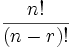Welcome

It is highly recommended that you have Javascript enabled; many features will not work unless you do. The recommended browsers are Firefox and Chrome; the board is also NOT mobile-friendly.

If you were referred by someone (ex. me, Jessica) please put their username in the referral box on the registration page. Ask them if you don't know their username.

If you are visiting for TESTING PURPOSES ONLY, this is the test account information:

Test

Select a forum to post in:

## Search found 1 match

### Combinations and Permutations

If the order doesn't matter, it is a Combination.
If the order does matter it is a Permutation.

PERMUTATIONS
Two types:
• Repetition is Allowed: a lock. It could be "333".
When you have n things to choose from, you have n choices each time.
When choosing r of them, the permutations are: n × n × ... (r times) = nr (this is the formula)
• No Repetition: first three people in a running race. You can't be first and second.
You have to reduce the number of available choices each time.
For example, what order could 16 pool balls be in? After choosing, say, number "14" you can't choose it again.
So, your first choice would have 16 possibilites, and your next choice would then have 15 possibilities, then 14, 13, etc. And the total permutations would be: 16 × 15 × 14 × 13 × ... = 20,922,789,888,000

But maybe you don't want to choose them all, just 3 of them, so that would be only: 16 × 15 × 14 = 3,360

Mathematically, we use the factorial function.
The factorial function (symbol: !) just means to multiply a series of descending natural numbers. Examples:
• 4! = 4 × 3 × 2 × 1 = 24
• 7! = 7 × 6 × 5 × 4 × 3 × 2 × 1 = 5,040
• 1! = 1
Note: it is generally agreed that 0! = 1. It may seem funny that multiplying no numbers together gets you 1, but it helps simplify a lot of equations.

So, if you wanted to select all of the billiard balls the permutations would be: 16! = 20,922,789,888,000
But if you wanted to select just 3, then you have to stop the multiplying after 14. How do you do that? There is a neat trick ... you divide by 13! ... = 16 × 15 × 14 = 3,360

Formula is:January 8th, 2013, 3:04 pm

Forum: Math Topics
Topic: Combinations and Permutations
Replies: 0
Views: 2,600